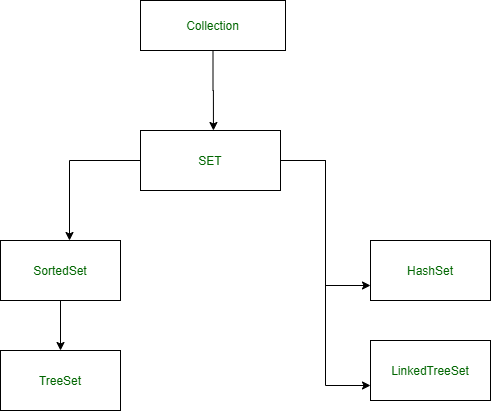Related Articles

# Convert Array to HashSet in Java

• Last Updated : 28 Dec, 2020

The Single Data structure cannot be able to fulfill the requirements of the programmers that’s why there are a lot of inbuilt Data-Structures in Programming languages.

Arrays are the most commonly used Data-Structure in most programming languages. The advantage of this Data-Structure is O(1) accessing the elements of the Arrays with the help of indexing but the most common disadvantages are we cannot change the size of the array after creating and the deletion of the elements is a complicated process in Arrays.

Sets: In Java, Any group of individual objects which are represented as a single unit is known as the collection of the objects. In Java, a separate framework named the “Collection Framework” has been defined in JDK 1.2 which holds all the collection classes and interface.  Sets are classified into two parts sorted set and unsorted set bot have advantage and disadvantage. Sorted Set i.e TreeSet sort its unique elements but the time-complexity of TreeSet is O(N log N) but unsorted Sets such as HashSet and LinkedSet do change the order of the elements but the difference between the HashSet and LinkedSet is Random Order of its elements.Examples:

```Input : Array: [1, 2, 3, 4, 5, 6]
Output: Set: [1, 2, 3, 4, 5, 6]

Input : Array: [a, b, c, d]
Output: Set: [a, b, c, d]```

Approach: Brute Force or Naive Method

Create an empty set  (HashSet if unsorted elements require) Iterate the elements of the array and add one by one to the set.

Example:

## Java

 `// Convert array to HashSet in Java``import` `java.io.*;``import` `java.util.Iterator;``// Importing Set libraries``import` `java.util.Set;``import` `java.util.HashSet;`` ` `class` `GFG {``    ``// Function to convert array to set``    ``static` `Set convert(``int``[] array)``    ``{``        ``// Hash Set Initialisation``        ``Set Set = ``new` `HashSet<>();`` ` `        ``// Iteration using enhanced for loop``        ``for` `(``int` `element : array) {``            ``Set.add(element);``        ``}``        ``// returning the set``        ``return` `Set;``    ``}`` ` `    ``// Function to print the set``    ``static` `void` `print(Set Set)``    ``{``        ``// Implement to iterator the Set``        ``Iterator _iterator = Set.iterator();`` ` `        ``// Iterate the elements of Set``        ``while` `(_iterator.hasNext()) {``            ``// print the element of the Set``            ``System.out.print(_iterator.next() + ``" "``);``        ``}``    ``}``    ``public` `static` `void` `main(String[] args)`` ` `    ``{`` ` `        ``// Array taken for consideration``        ``int` `array[] = { ``1``, ``2``, ``3``, ``4``, ``5``, ``6` `};`` ` `        ``// Calling function to convert the array``        ``Set Set = convert(array);`` ` `        ``// print the set``        ``print(Set);``    ``}``}`
Output
`1 2 3 4 5 6`

Approach 2

Using Java 8 Stream API: HashSet constructor can take another collection object to construct a new set containing the elements of the specified array.

1. Get the Array to be converted.
2. Convert the array to Stream
3. Convert the Stream to Set using Collectors.toSet()
4. Collect the formed set using the collect() method
5. Return the formed Set.

Example:

## Java

 `// Convert Array to HashSet in Java`` ` `import` `java.util.*;``import` `java.util.stream.*;`` ` `class` `GFG {`` ` `    ``// Generic function to convert array to set``    ``public` `static` ` Set convertArrayToSet(T array[])``    ``{``        ``// create a set from the Array``        ``return` `Arrays.stream(array).collect(``            ``Collectors.toSet());``    ``}`` ` `    ``public` `static` `void` `main(String args[])``    ``{``        ``// Create an Array``        ``String array[]``            ``= { ``"Geeks"``, ``"forGeeks"``, ``"A computer Portal"` `};`` ` `        ``// Print the Array``        ``System.out.println(``"Array: "``                           ``+ Arrays.toString(array));`` ` `        ``// convert the Array to Set``        ``Set set = convertArrayToSet(array);`` ` `        ``// Print the Set``        ``System.out.println(``"Set: "` `+ set);``    ``}``}`
Output
```Array: [Geeks, forGeeks, A computer Portal]
Set: [A computer Portal, Geeks, forGeeks]```

Attention reader! Don’t stop learning now. Get hold of all the important Java Foundation and Collections concepts with the Fundamentals of Java and Java Collections Course at a student-friendly price and become industry ready. To complete your preparation from learning a language to DS Algo and many more,  please refer Complete Interview Preparation Course.

My Personal Notes arrow_drop_up